Courses

# Test: Ratio And Proportion- 3

## 15 Questions MCQ Test Quantitative Aptitude for GMAT | Test: Ratio And Proportion- 3

Description
This mock test of Test: Ratio And Proportion- 3 for Quant helps you for every Quant entrance exam. This contains 15 Multiple Choice Questions for Quant Test: Ratio And Proportion- 3 (mcq) to study with solutions a complete question bank. The solved questions answers in this Test: Ratio And Proportion- 3 quiz give you a good mix of easy questions and tough questions. Quant students definitely take this Test: Ratio And Proportion- 3 exercise for a better result in the exam. You can find other Test: Ratio And Proportion- 3 extra questions, long questions & short questions for Quant on EduRev as well by searching above.
QUESTION: 1

### The difference between the two positive numbers is 10 and the ratio between them is 5:3. Find the product of the two numbers.

Solution:

Let the nos. be 5x and 3x. then, 5x - 3x = 10 2x = 10, x = 5 Required product = 5x x 3x = 5 x 5 x 3 x 5 = 375

QUESTION: 2

### Four numbers in the ratio of 1:3:4:7 add up to give a sum of 75. Find the value of the biggest number.

Solution:

Let the number be x, 3x, 4x and 7x. then, x + 3x + 4x + 7x = 75 15x = 75 ⇒ x = 5 Required value = 7 x 5 = 35

QUESTION: 3

### According to the Boyle’s law, at a constant temperature, pressure of a definite mass of gas is inversely proportional to the volume. If the pressure is reduced by 20%, find the respective change in volume.

Solution: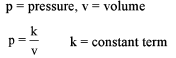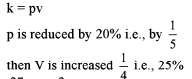QUESTION: 4

P is directly proportional to Q, and Q = 7 when P = 15, find P when Q = 14.

Solution: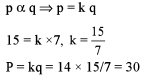QUESTION: 5

If p/q = 3/4, then find the value of the expression (5p - 3q)/(7p + 2q).

Solution: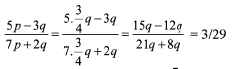QUESTION: 6

A and B invested Rs 12,000 and Rs 18,000 respectively in a business for the whole year. At the year-end, there was a total profit of Rs 2,000. What is the share of A in the profit?

Solution:

Ratio of their investment = 12000 : 18000 = 2: 3 A’s share in profit = (2/5) x 2000 = Rs 800

QUESTION: 7

A and B invest Rs 12,000 and Rs 16,000 respectively in a business. At the year-end, they share the profit in the ratio of 3:1. If A has invested his capital for the whole year, for how many months B has invested his capital?

Solution:

Let B invested for n months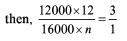n = 3

QUESTION: 8

The sum of the ages of the 4 members of Sinha family is 140 years. 5 years ago the ages of the 4 members Nishu, Vicky, Mrs Sinha and Mr Sinha were in the ratio of 2 : 3 : 7 : 8 . After how many years would Nishu be as old as the present age of his mother?

Solution:

Let their ages 5 years ago be 2x, 3x, 7x and 8x. Their ages now 2x + 5, 3x + 5, 7x + 5, 8x + 5.

Or, 20x + 20 = 140 ⇒ 2Ox = 120 ⇒ x = 6

Present age o f Nishu = 2 x 6 + 5 = 17 years

Present age o f mother = 7 x 6 + 5 = 47 years

Hence, required years (47 -17) years = 30 years

QUESTION: 9

Two-fifth of Anil’s salary is equal of Bhuvan’s salary and seven -ninth of Bhuvan’s salary is equal to Chandra’s salary. The sum of the salary of all of them is Rs 770. Which of the following is the salary of each one of them?

Solution: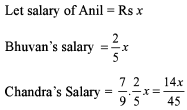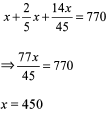QUESTION: 10

Bidhan is planning to buy a bike worth Rs 35,000, provided his brother agrees to lend him 3/2 times the money Bidhan contributes and the financer provides 3 times the brother’s contribution. How much does Bidhan’s brother contribute?

Solution: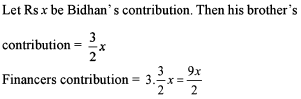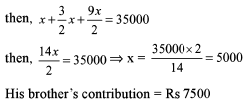QUESTION: 11

An alloy of manganese, tin and bronze contains 90% bronze, 7% manganese and 3% tin. A second alloy of bronze and tin is melted with the first and the mixture contains 85% of bronze, 5% of manganese and 10% of tin. What is the percentage of bronze in the second alloy?

Solution: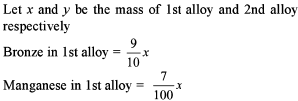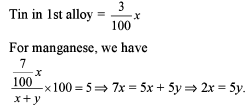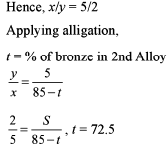QUESTION: 12

Two cogged wheels of which one has 32 cogs and other 54 cogs, work into each other. If the latter turns 80 times in three quarter of a minute, how often does the other turn in 8 seconds? (Assume equal size cogs and equi-spaced).

Solution: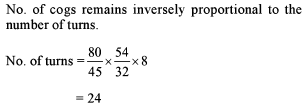QUESTION: 13

A bag contains Rs 600 in the form of one rupee, 50 paise and 25 paise coins in the ratio of 3:4:12. Find the total number of 25 paise coins in the bag.

Solution: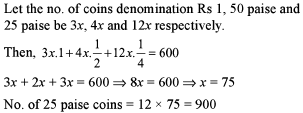QUESTION: 14

Anil started a manufacturing unit with a certain amount of money. After a few months, Dheeraj became his partner, contributing three times of what Anil had contributed. At the end of the year, each was entitled to half the total profit. If Anil started the unit in January, then when did Dheeraj join as a partner?

Solution:

Let Anil invested Rs X , then D heeraj’s investment = Rs 3x Let Dheeraj join him for n month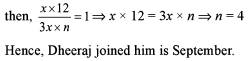QUESTION: 15

In a fort there are 1600 soldiers fighting a battle against their enemy. On a particular day after their morning meal the balance provision inside the fort is only sufficient for 12 days at the rate of 1.2 kg per day. If by the evening 400 soldiers die then for how many days will the provision be sufficient for remaining men at the rate of 1.6 kg per day. (Assume soldiers eat either in morning or in evening).

Solution:

Total quantity of food at the beginning of the day
= (1600) (12) (1.2)
Total number of soldiers left at the end of the day 1200
Now we have to calculate that for how long is available provision enough for the left-out soldiers
(1200) (x) (1.6)
(1600) (12) (1.2) = (1200) (x) (1.6)
Solving it for the value of x
We get x = 12
The provision is sufficient for 1200 soldiers for 12 days.